# 北方沿海路基土工程特性试验研究Experimental Study on the Characteristics of Subsoil Engineering in Northern Coastal Roadbed

DOI: 10.12677/HJCE.2018.73039, PDF, HTML, XML, 下载: 667  浏览: 872  国家自然科学基金支持

Abstract: Because the north coastal embankment environment is complex, with specific design and con-struction beyond the status quo of the standard and experience, research on the northern coastal related properties of subgrade soil has important engineering significance. A series of geotechnical tests were carried out for the northern coastal subgrade soil, and a series of indicators reflecting the physical and mechanical properties of soil were measured. The research shows that some northern coastal embankments are coarse grained soils with high water content, low shear strength, good permeability, poor compressibility and fast consolidation. It can provide the basis for the improvement and reinforcement of the northern coastal subgrade soil, and also the calculation of the settlement of the northern coastal subgrade and the calculation of the bearing capacity of the subgrade.

1. 引言

2. 土工试验及土的各项指标

2.1. 土的含水率

$\omega =\frac{m-{m}_{\text{s}}}{{m}_{\text{s}}}×100$ (1)

2.2. 土的界限含水率

A线方程 ${I}_{\text{P}}=0.73×\left({\omega }_{\text{L}}-20\right)$ B线方程 ${\omega }_{\text{L}}$ = 50%Table 1. Liquid limit plastic limit joint test record table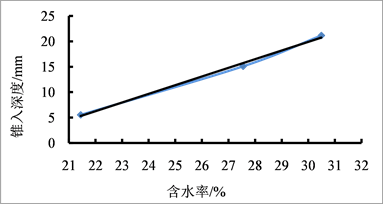Figure 1. Diagram of the relation between the depth of the cone penetration and the water content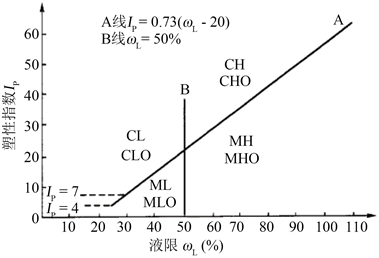Figure 2. Plastic diagram

${I}_{\text{P}}=0.73×\left({\omega }_{\text{L}}-20\right)$ (2)

${I}_{\text{P}}=0.73×\left(30.1-20\right)=7.373<9.43$

2.3. 颗粒级配分析试验

${C}_{\text{u}}=\frac{{d}_{60}}{{d}_{10}}$ (3)

${C}_{\text{c}}=\frac{{d}_{30}^{2}}{{d}_{60}{d}_{10}}$ (4)Table 2. Particle analysis results2.4. 最佳含水率试验

${m}_{\omega }=\frac{m}{1+0.01{\omega }_{0}}×0.01\left(\omega -{\omega }_{0}\right)$ (5)

$\omega =\left(\frac{m}{{m}_{\text{d}}}-1\right)×100$ (6)

${\rho }_{\text{d}}=\frac{\rho }{1+0.01\omega }$ (7)

5个试件分别以含水率为横坐标，干密度为纵坐标，绘制含水率与干密度的关系曲线如图4，取干密度最大点对应的含水率值为最佳含水率，对应纵坐标值为最大干密度，从数据曲线中得出该土样的最佳含水率为20.5%，最大干密度1.680 g/cm3

2.5. 渗透性试验

${k}_{\text{t}}=2.3\frac{aL}{A\left({t}_{2}-{t}_{1}\right)}\mathrm{lg}\frac{{h}_{1}}{{h}_{2}}$ (8)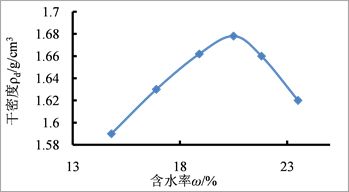Figure 4. Relationship between ρd~ω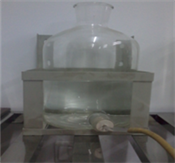(a) 供水瓶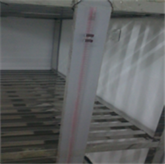(b) 变水头管

Figure 5. Variable head test device

2.6. 直剪试验

1) 对准剪切容器上下盒，插入固定销，在下盒内放透水石和滤纸，将带有试样的环刀刃向上，对准剪盒口，在试样上放滤纸和透水石，将试样小心地推入剪切盒内。

2) 移动传动装置，使上盒前端钢珠刚好与测力计接触，依次加上传压板、加压框架，安装垂直位移量测装置，测记初始读数。

3) 根据工程实际和土的软硬程度施加各级垂直压力，然后向盒内注水。当试样为非饱和试样时，应在加压板周围包以湿棉花。Table 3. Data of variable head permeability test results

4) 施加垂直压力，垂直压力的大小应根据工程要求和土的软硬状态确定，宜按25 kpa、50 kpa、100 kpa、200 kpa或100 kpa、200 kpa、300 kpa、400 kpa施加压力。拔出固定销立即开动秒表，以小于0.02 mm/min的剪切速度进行。

5) 当测力计百分表读数不变或后退时，继续剪切至剪切位移为4 mm时停止，记下破坏值。当剪切过程中测力计百分表无峰值时，剪切至剪切位移达6 mm时停止。

6) 剪切结束，吸去盒内积水，退剪切力和垂直压力，移动压力框架，取出试样，测定含水率。

$\Delta L=20n-R$ (9)

$\tau =CR$ (10)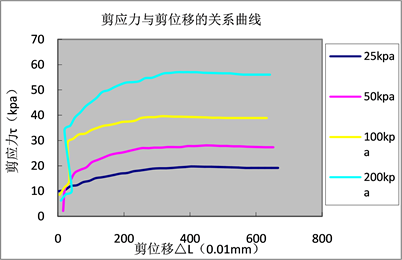Figure 6. Relationship of shear stress vs. shear displacement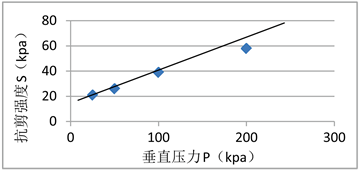Figure 7. Relationship between shear strength s and vertical pressure p

2.7. 土的固结实验

${e}_{\text{i}}={e}_{0}-\left(1+{e}_{0}\right)\frac{\Delta {h}_{\text{i}}}{{h}_{0}}$ (11)

${a}_{\text{v}}=\frac{{e}_{\text{i}}-{e}_{\text{i}+\text{1}}}{{p}_{\text{i}+\text{1}}-{p}_{\text{i}}}$ (12)

${E}_{\text{s}}=\frac{1+{e}_{0}}{{a}_{\text{v}}}$ (13)

${m}_{\text{v}}=\frac{1}{{E}_{\text{s}}}=\frac{{a}_{\text{v}}}{1+{e}_{0}}$ (14)

${C}_{\text{c}}或{C}_{\text{s}}=\frac{{e}_{\text{i}}-{e}_{\text{i}+\text{1}}}{\mathrm{lg}{p}_{\text{i}+\text{1}}-\mathrm{lg}{p}_{\text{i}}}$ (15)

${C}_{\text{v}}=\frac{0.848{\left(\stackrel{¯}{h}\right)}^{2}}{{t}_{90}}$ (16)

$\stackrel{¯}{h}=\frac{{h}_{1}+{h}_{2}}{4}$ (17)

$\stackrel{¯}{h}$ ——最大排水距离，等于某压力下试样初始与终了高度的平均值之半，cm。

${t}_{90}$ ——固结度达到90%所需时间s。

3. 小结

1) 部分北方沿海路基土属于粗粒土，含水量高，试验粘土天然含水率为20%，最佳含水率为20.5%，液限为30.1%，塑限为20.67%，塑性指数为9.43%，不均匀系数为8.5，曲率系数为1.86，最大干密度1.680 g∙cm−3，黏土的干密度为1.604 g∙cm−3。可为北方沿海路基土进行沉降计算提供数据参考。Table 4. Compression index under various pressuresTable 5. Coefficient of consolidation under various pressures

2) 部分北方沿海路基土抗剪强度低，透水性好，压缩性差，固结速度较快。可为沿海路基土的改良、加固等提供参考。

  江宗斌. 沿海路基变形特性试验与数值模拟研究[D]: [硕士学位论文]. 大连: 大连海事大学, 2013.  刘怡林, 黄茂松. 公路路基地基承载特性及破坏模式[J]. 地下空间与工程学报, 2010, 6(1): 84-89.  陈明珠, 阎长虹, 王玉英, 詹启伟, 郭军辉, 郑军, 陈喜. 土体渗透性影响因素——以普定陈旗堡为例[J]. 水文地质工程地质, 2008(4): 66-70.  JTG E40-2007. 公路土工试验规程[S].  刘红军. 土质学与土力学[M]. 北京: 北京大学出版社, 2013.  龚晓楠. 高等级公路地基处理设计指南[M]. 北京: 人民交通出版社, 2015: 4-5.  卢廷浩. 土力学[M]. 北京: 高等教育出版社, 2010.  松岡元. 土力学[M]. 罗汀, 姚仰平, 编译. 北京: 中国水利水电出版社, 2001.